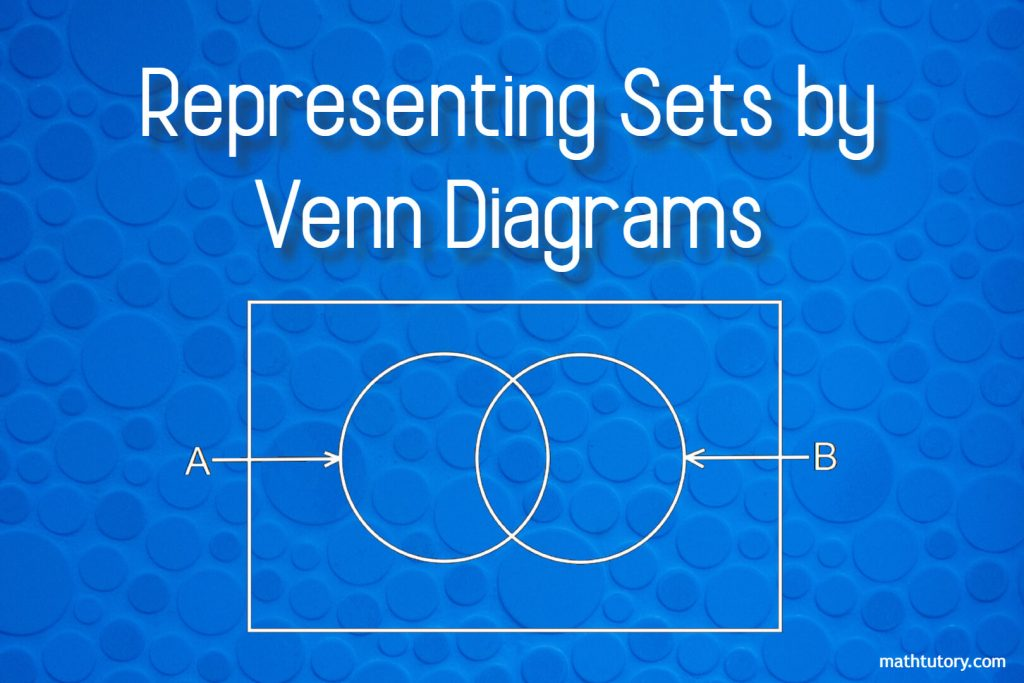## Representing Sets by Venn Diagrams

Venn diagrams are the most common way of representing a set. This method was introduced by the English mathematician John Venn. Therefore these are called Venn Diagram. Set is a collection of items that can be clearly identified.The items in a set are called its elements. Example: Let A be the set of even numbers …